# Linear system of differential equations with almost-periodic coefficients

(diff) ← Older revision | Latest revision (diff) | Newer revision → (diff)

A system of ordinary differential equations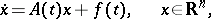(1)

where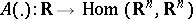,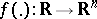are almost-periodic mappings (cf. Almost-periodic function). In coordinate form one has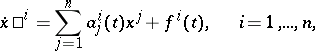where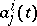and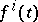,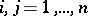, are almost-periodic real-valued functions. Such systems arise in connection with Bohr almost-periodic functions (see ). Interest in a narrower class of systems (where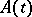andare quasi-periodic mappings, cf. Quasi-periodic function) arose much earlier in connection with the examination of variational equations along conditionally-periodic solutions of the equations of celestial mechanics.

If the homogeneous system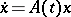(2)

is a system with integral separation (see Integral separation condition), then it reduces to a diagonal system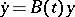with almost-periodic coefficients by an almost-periodic (with respect to) Lyapunov transformation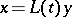; that is, it reduces to a system for which there is a basis of, independent of, consisting of vectors that are eigen vectors of the operator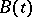for every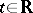. In coordinates with respect to this basis the systemis written in diagonal form: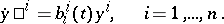The set of systems with integral separation is open in the space of systems (2) with almost-periodic coefficients, endowed with the metric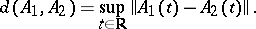The following theorem holds. Let, where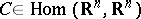, let the eigen values ofall be real and distinct, and let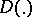be an almost-periodic mapping. Then there is an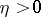such that for allwith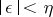the system (2) reduces to a diagonal system with almost-periodic coefficients, by an almost-periodic (with respect to) Lyapunov transformation.

For an almost-periodic mapping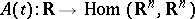the following four assertions are equivalent: 1) for every almost-periodic mappingthere is an almost-periodic solution of the system (1); 2) there is exponential dichotomy of solutions of the system (2); 3) none of the systems, where, has non-zero bounded solutions; and 4) for every bounded mapping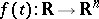there is a bounded solution of the system (1).

How to Cite This Entry:
Linear system of differential equations with almost-periodic coefficients. Encyclopedia of Mathematics. URL: http://encyclopediaofmath.org/index.php?title=Linear_system_of_differential_equations_with_almost-periodic_coefficients&oldid=17504
This article was adapted from an original article by V.M. Millionshchikov (originator), which appeared in Encyclopedia of Mathematics - ISBN 1402006098. See original article